[BACK]Computer Modeling in Engineering & SciencesDOI: 10.32604/cmes.2022.019140

ARTICLE

A Novel Method for the Reconstruction of Road Profiles from Measured Vehicle Responses Based on the Kalman Filter Method

1School of Automation, Northwestern Polytechnical University, Xi’an, 710072, China
2School of Mechanics, Civil Engineering & Architecture, Northwestern Polytechnical University, Xi’an, 710072, China
3Chinese Flight Test Establishment, Xi’an, 710089, China
*Corresponding Author: Xu Long. Email: xulong@nwpu.edu.cn
Received: 5 September 2021; Accepted: 29 September 2021

Abstract: The estimation of the disturbance input acting on a vehicle from its given responses is an inverse problem. To overcome some of the issues related to ill-posed inverse problems, this work proposes a method of reconstructing the road roughness based on the Kalman filter method. A half-car model that considers both the vehicle and equipment is established, and the joint input-state estimation method is used to identify the road profile. The capabilities of this methodology in the presence of noise are numerically demonstrated. Moreover, to reduce the influence of the driving speed on the estimation results, a method of choosing the calculation frequency is proposed. A road vibration test is conducted to benchmark the proposed method.

Keywords: Road profile reconstruction; inverse problems; precise integration; Kalman filter; spatial resolution

1  Introduction

The road profile is considered an essential input that affects the vehicle dynamics; it may lead to the fatigue failure of the vehicle components or worsened riding comfort, especially for some special delivery vehicles. The understanding of vehicle responses is important for road quality evaluation, road roughness index calculation, vehicle dynamics analysis, suspension design, and control system development . For technical and economic reasons, this information cannot be measured in standard vehicles, and must therefore be estimated using unique methods. The estimation of the excitation acting on a system from a given response is a typical inverse problem, which is usually ill-posed.

To ensure the normal usage, measurement, and maintenance of the road, a number of profilometers have been developed. Longitudinal profile analyzer (LPA) profilometers are tools and methods used to generate numerical sequences related to real road profiles [2,3]. However, the major drawback of these profilers is that they are not economical enough for application in common vehicles. There also exist some profile measurement methods based on visual inspections , but these are extremely expensive and may be impossible to apply in rainy weather. Some scholars have used neural networks to establish correlations between the vehicle response and road roughness, but they were revealed to be too complicated and time-consuming . To deal with these problems, a method of control constraints was proposed by Burger ; however, this method is more suitable for nonlinear and complex models. An algebraic estimator designed for the estimation of the road profile excitation was investigated by Haddar et al. , and was validated on a quarter model with two degrees of freedom (DOFs).

While some methods based on model-based sliding mode observers were proposed in recent studies [4,10], the researchers considered a complex model, which is time-consuming. The final reconstruction result heavily relies on the quality of the model. Because this is an inverse problem in mechanics, relevant methods in this field can be referenced. In recent years, some deterministic-stochastic methods have been developed; these methods consider noise as a random process and assume that noise is involved not only in measurements, but also in state variables. Gillijns et al.  developed a recursive filter that uses system outputs to estimate system inputs and states. An augmented Kalman filter was proposed by Lourens et al.  for force recognition in structural dynamics; this filter includes the unknown force in the state vector and estimates it in combination with the state. Maes et al. [13,14] proposed a joint input-state estimation algorithm that can be used to identify the forces applied to structures, and can extrapolate the measured data to the unmeasured response quantity of interest. In addition, because it is constructed in a spatial state, it is ideal for combining information from different sensors available in the vehicle. Fauriat et al.  used an algorithm based on Kalman filtering theory to estimate the profiles of a road covered by a given vehicle.

The objective of the present research was to design, test, and apply an estimation algorithm to predict road roughness information from the measured response of a heavy special vehicle based on a half-car model. The remainder of this paper is organized as follows. First, road profile identification is defined as a typical inverse problem in mathematical physics based on a half-vehicle model in state space. Second, a method based on the principle of the selected algorithm, namely a joint input-response estimation method, is proposed to solve inverse problems within the stochastic framework. Then, a structural model of a heavy special vehicle at different speeds is considered as an example to verify the effectiveness of the proposed algorithm. Finally, several conclusions are drawn, and directions for future work are proposed.

2  Dynamic Equations of the Vehicle

When transporting certain equipment, the middle line of the vehicle can be placed along its longitudinal symmetric surface, and the sway vibration is generally smaller; thus, the model shown in Fig. 1 can be reduced to a two-dimensional plane model.

There are six DOFs in the model, which respectively represent the movement of the suspension, body, and equipment, and the coordinate system is constructed on the moving vehicle. The tire is linked with the road displacement u(t), which involves the tire’s stiffness kt. The tire damping is assumed to be negligible. The truck has a rear two-axle suspension, but for the convenience of calculation, the parameters at each axis are combined. Then, the following 6-DOF differential equations of motion can be obtained.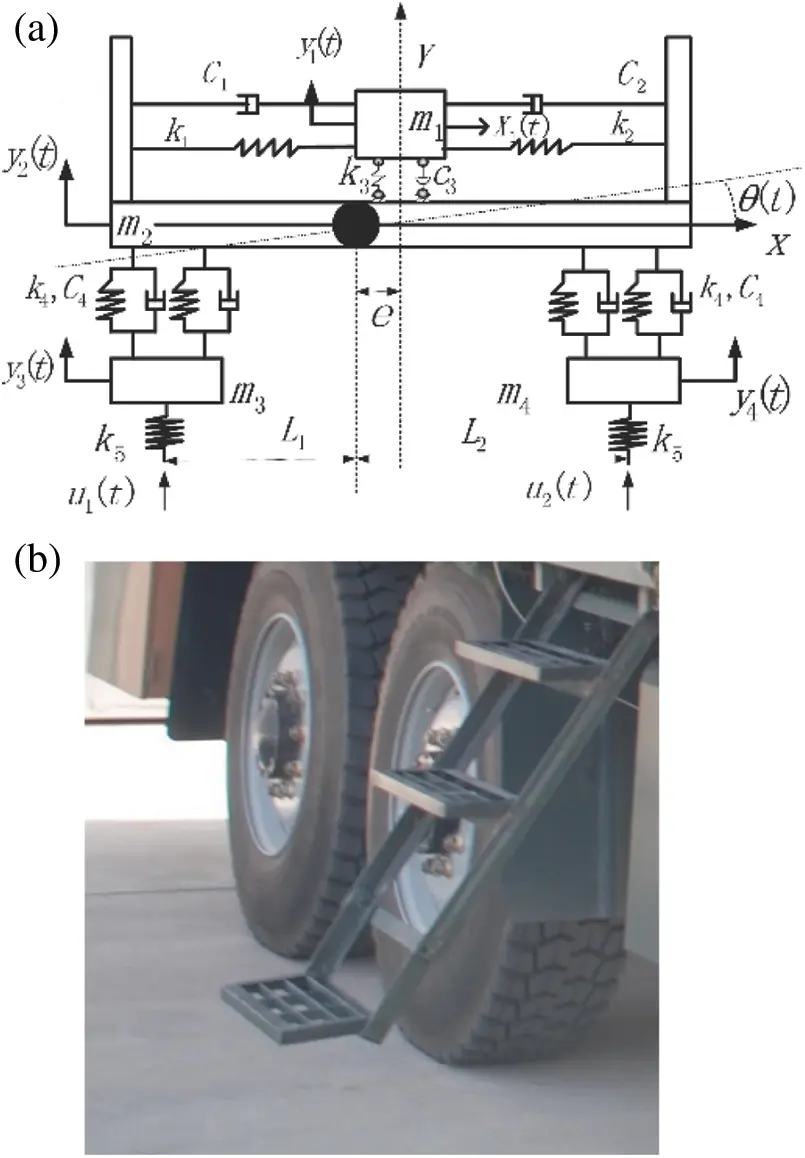Figure 1: The equipment and transport vehicle model. (a) Vehicle model (b) Two-axle suspension

m1x¨1(t)+(c1+c2)x˙1(t)+(k1+k2)x1(t)(c1L1+c2L2)2θ˙2(t)(k1L1+k2L2)2θ2(t)=0 (1)

m1y¨1(t)+c3y˙1(t)c3y˙2(t)ec3θ˙(t)+k3y1(t)k3y2(t)k3eθ(t)=0 (2)

m2y¨2(t)c3y˙1(t)+(4c4+c3)y˙2(t)2c4y˙3(t)2c4y˙4(t)+(ec3+2c4L22c4L1)θ˙(t)k3y1(t)+(k3+4k4)y2(t)2k4y3(t)2k4y4(t)+(ek32L1k4+2k4L2)θ(t)=0 (3)

m3y¨3(t)2c4y˙2(t)+2c4y˙3(t)+2c4L1θ˙(t)2k4y2(t)+(2k4+k5)y3(t)+2k4L1θ(t)=k5u1(t) (4)

m4y¨4(t)2c4y˙2(t)+2c4y˙4(t)2c4L2θ˙(t)2k4y2(t)+(2k4+k5)y4(t)2k4L2θ(t)=k5u2(t) (5)

Iθ¨(t)ec3y˙1(t)+(2L2c4+ec32c4L1)y˙2(t)+2c4L1y˙3(t)2c4L2y˙4(t)+(2c4L12+2c4L22+e2c3)θ˙(t)ek3y1(t)+(ek3+2k4L22k4L1)y2(t)+2k4L1y3(t)2k4L2y4(t)+(2L12k4+e2k3+2L22k4)θ(t)=0 (6)

Eqs. (1)(6) constitute a 6-DOF nonlinear coupled dynamical differential equation system. Eq. (1) includes the nonlinear coupling terms θ2(t) and θ˙2(t) in the body pitching angle θ. Because the length of the vehicle body was about 10 m, the pitch motion of the vehicle was much smaller than the vertical vibration of the body during the actual driving test; thus, the second-order traces θ2(t) and θ˙2(t) can be ignored. Therefore, Eq. (1) is not coupled with Eqs. (2)(6), so it can be solved separately. During the study of the vibration of vehicles and equipment, the solution and calculation of Eqs. (2)(6) can be considered. The parameters and physical significance of the vehicle and equipment model are exhibited in Table 1.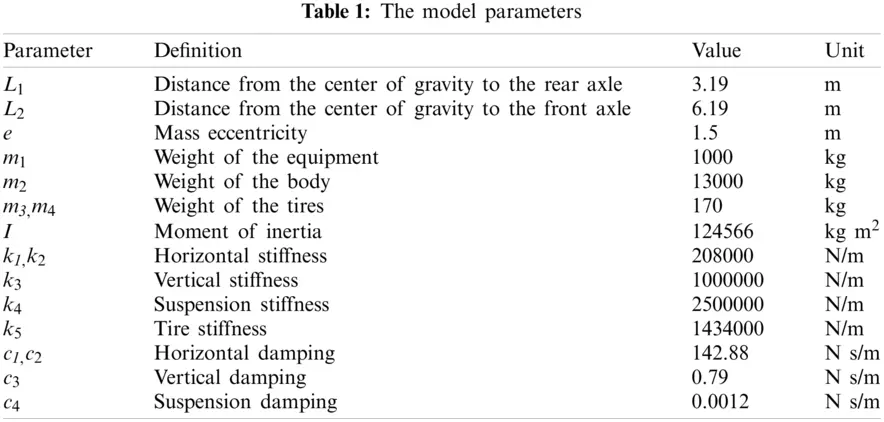Eqs. (2)(6) can be written in matrix form as Eq. (7):

MY¨+CY˙+KY=SpF(t), (7)

where M, C, and K respectively denote the mass, damping, and stiffness matrices, and Sp denotes the input force influence matrix, each column of which provides the spatial distribution of the load time history in the corresponding element of the excitation vector F(t).

The following matrices are defined for the model:

M=[m100000m200000m300000m400000I],C=[c3c300ec3c34c4+c32c42c4(ec3+2c4L22c4L1)02c42c402L1c402c402c42L2c4ec3(ec3+2c4L22c4L1)2L1c42L2c4(2c4L12+2c4L22+e2c3)],K=[k3k300ek3k3(k3+4k4)2k42k4(ek3+2L2k42L1k4)02k4(2k4+k5)02L1k402k40(2k4+k5)2L2k4ek3(ek3+2L2k42L1k4)2L1k42L2k4(e2k3+2L12k4+2L22k4)],Y¨={y¨1y¨2y¨3y¨4θ¨};Y˙={y˙1y˙2y˙3y˙4θ˙};Y={y1y2y3y4θ}, Sp=, and F=[k5u1(t)k5u2(t)]

It is assumed that the front and rear wheels of the vehicle are driving in the same line; thus, the road excitations u1(t) and u2(t) are the same, but there is a time delay between them. The relationship between u1(t) and u2(t) in the time domain is given by the following equation:

u1(t)=u2(tΔt),Δt=L1+L2v (8)

3  Mathematical Formulation

By introducing the state vector X(t)=[Y(t)Y˙(t)], Eq. (7) can be written as

X˙(t)=AX(t)+BF(t), (9)

where the system matrices A and B are defined as

A=[0n×nIn×nM1KM1C]2n×2n, B=[0n×nM1Sp]2n×1.

Consider the measurement data vector Z(t), which is expressed as a linear combination of the displacement, velocity, and acceleration vectors, as follows:

Z(t)=SaY¨(t)+SvY˙(t)+SdY(t), (10)

where Sa, Sv, and Sd are selection matrices for acceleration, velocity, and displacement, respectively. Eq. (12) can be transformed into its state-space form:

Z(t)=GX(t)+JF(t), (11)

where the output influence matrix and direct transmission matrix are defined as

G=[SdSaM1KSvSaM1C], J=[SaM1Sp].

The state-space model is derived starting from the classical discrete-time state equation with unknown noise vectors wk and vk which respectively represent the stochastic system and measurement noise.

Xk+1=AcXk+BcFk+wk, (12)

Zk=GXk+JFk+vk, (13)

where Xk=X(kΔt), Fk=F(kΔt), Zk=Z(kΔt), k=1,,N), Ac=exp(AΔt), and Bc=[AcI]A1B. Moreover, and wk and vk are assumed to be mutually uncorrelated white noise signals with known covariance matrices Q=E{wkwlT}0 and R=E{vkvlT}>0. To maintain the stability of the calculation, Ac can be solved by using the precise integration method .

A generic Kalman filter can be defined as a recursive linear state estimator designed to be optimal in a minimum-variance unbiased sense. A state estimate X^k|l is defined as an estimate of Xk given {Zn}n=0l and its error covariance matrix Pk|l as E[ (XkX^kl)(XkX^kl)T ].

In this context, the recursive prediction scheme can be applied to the measurement data Zk, from which the excitation is extracted. The initialized conditions X^0|1 and F~0|1 are assumed to exist. The excitation and state estimates are then computed by following Steps (a) to (c).

(a)   Input estimation

R~k=GPk|k1GT+RMk=(JTR~k1J)1JTR~k1F^[k|k]=Mk(ZkGX^[k|k1])PF[k|k]=(JTR~k1J)1

(b)   Measurement update

Lk=Pk|k1GTR~k1X^[k|k]=X^[k|k1]+Lk(ZkGX^[k|k1]JF^[k|k])Pk|k=Pk|k1Lk(R~kJPF[k|k]JT)LkTPXF[k|k]=PXF[k|k]T=LkJPF[k|k]

(c)   Time update

X^[k+1|k]=AcX^[k|k]+BcF^[k|k]Pk+1|k=[AcBc][Pk|kPXF[k|k]PXF[k|k]PF[k|k]][AcTBcT]+Q

In the present study, the state vector of the Kalman filter is X=[y˙1,y˙2,y˙3,y˙4,θ˙,y1,y2,y3,y4,θ]T, and the measured vector is Z=[y1,y2,y3,y4,θ]T. The model of the vehicle considered in this study is more complex than that which was considered in previous research , as the present model has more DOFs. This method is mainly used to estimate the input, but it can also be used to estimate the unmeasured response of the structure.

4  Simulation Results

In this section, the numerical results of the proposed estimator are presented. Two types of road profiles are considered, and the variation of the vehicle speed is taken into account to discuss the robustness of the estimators. Finally, the flowchart of the algorithm is provided.

A large amount of testing data indicates that road roughness is a stationary, Gaussian process with a zero mean and ergodic randomness, so a random input can reflect the actual road condition of the vehicle. Referring to the ISO8608 standard, the power spectral density (PSD) of pavement roughness can be fitted with the following formula:

Gq(n)=Gq(n0)(nn0)W, (14)

where n denotes the spatial frequency, which represents the number of cycles in which the wave is contained per meter, the unit of which is m−1; n0 = 0.1 m−1 denotes the reference spatial frequency. Moreover, Gq(n0) denotes the PSD of the pavement roughness under the reference spatial frequency, which depends on the road level; it is also called the road roughness coefficient. W = 2 is the frequency index, which is the frequency of the oblique line in the double logarithmic coordinate; it determines the frequency structure of the roughness PSD. White noise can be used to simulate the road surface roughness, and its time-domain description is given by Eq. (15):

u˙r(t)=2πn1vur(t)+2πn0Gq(n0)vω(t), (15)

where n1 = 0.1 m−1 denotes the lower cut-off spatial frequency, ω(t) denotes zero-mean white noise, ur(t) denotes the vertical displacement excitation, and v denotes the speed of the transport vehicle. The road profile in the space domain is presented in Fig. 2 (v = 10 m/s).Figure 2: The road profile of ISO type E

The response of the vehicle was calculated in MATLAB by the precise Runge-Kutta integration method. The vertical acceleration responses of the vehicle body, front wheel, and rear wheel at v = 10 m/s are presented in Fig. 3, and the sampling frequency of the simulation was 200 Hz.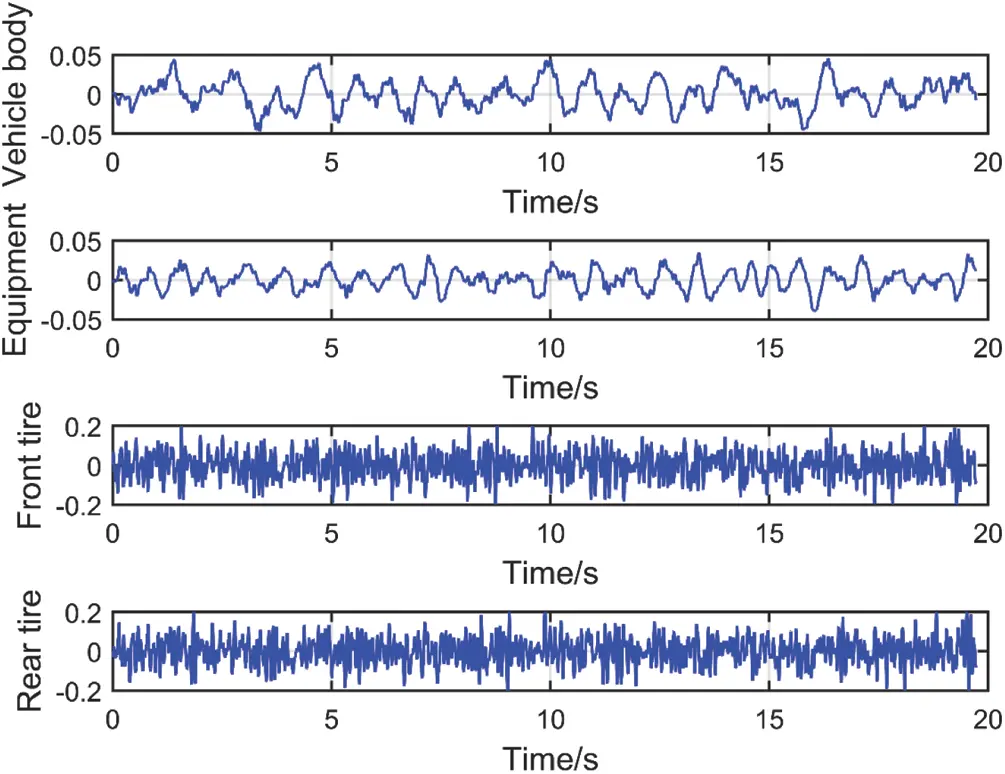Figure 3: The responses of the vehicle

An important feature of the proposed method is the determination of its parameters, including the covariances and initial values. An empirical assessment of the magnitude of the parameters proposed in a previous study was adopted in the present work . For a rough estimation of the parameters, the diagonal elements of the matrix Q were set as [1e41e41e21e21e41e81e81e71e71e8], and the diagonal elements of the matrix R were set as [1e41e41e21e21e4]. The initial presumptions of the diagonal element of P0|1 were all set as 5 × 10−5.

Once the parameters were determined, the proposed algorithm was used to estimate the road roughness, and the results are exhibited in Figs. 4 and 5. As can be seen from the figures, the road profile was identified comparatively accurately. It should be noted that the maximum standard spatial frequency was 10 m−1. To recover the time-domain signal without distortion, the sampling frequency should not be less than twice the highest frequency in the analog signal spectrum. When analyzing the time-domain model, the maximum spatial frequency corresponding to the maximum time frequency was 10 m−1, and only half of the maximum time frequency can be analyzed when analyzing the PSD of the signal (the corresponding spatial frequency was 5 m−1).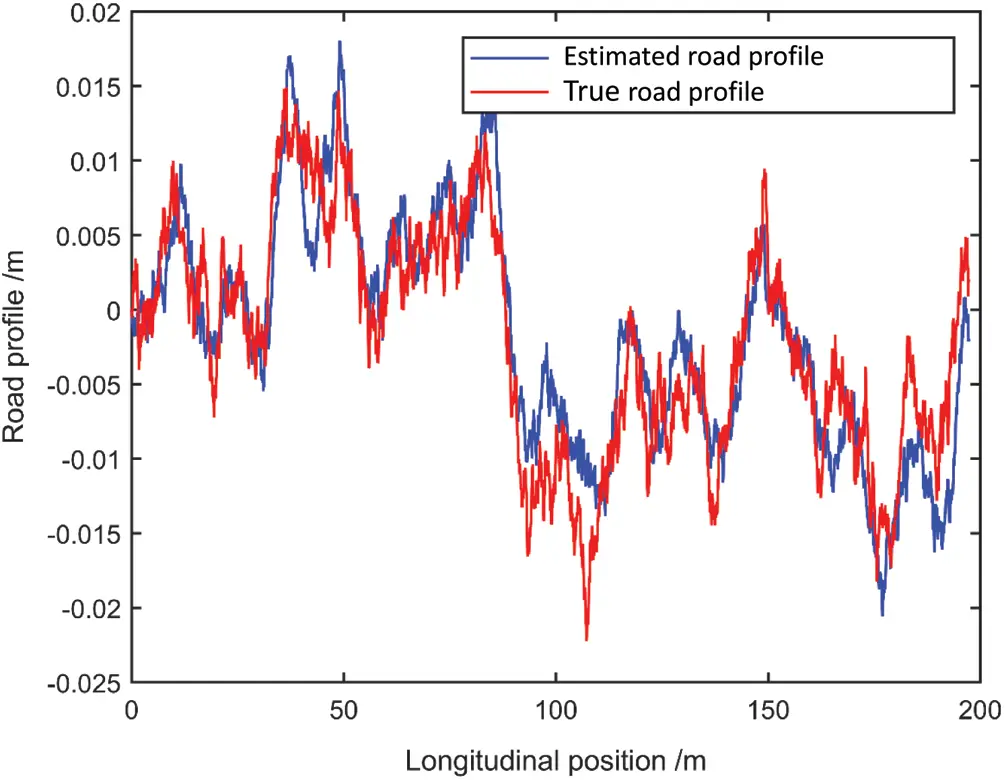Figure 4: The comparison of the estimated and true road profiles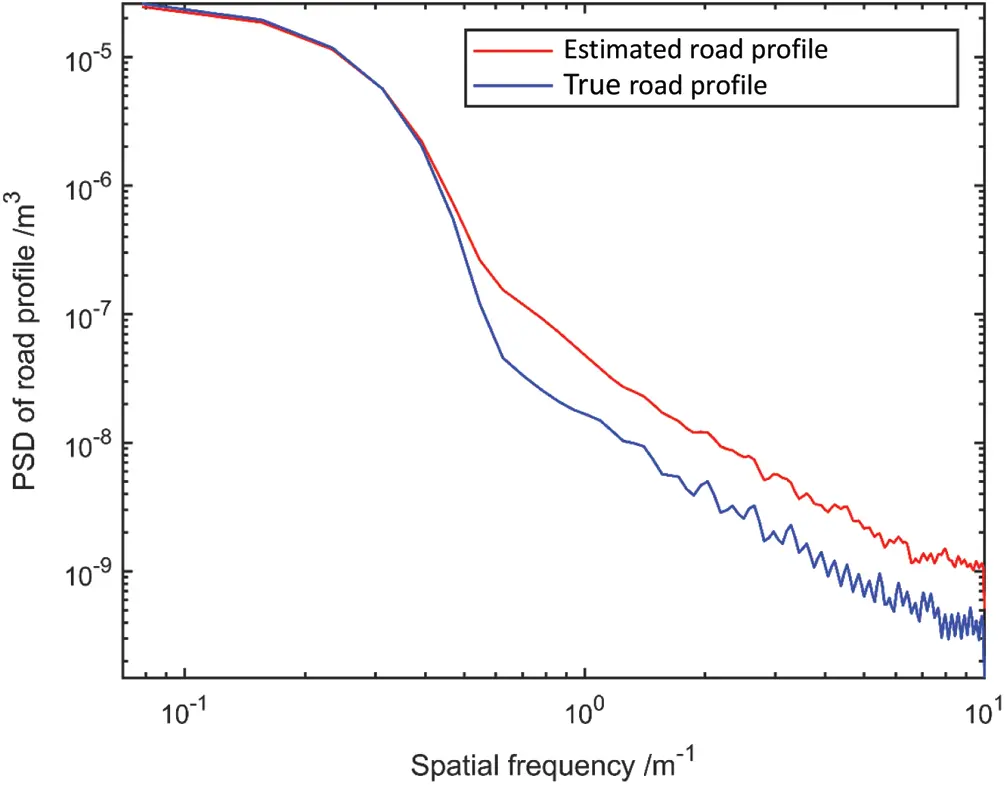Figure 5: The comparison of the estimated and true road profiles in terms of the PSD

Because the model is random, the results of each run were different. Table 2 presents the root-mean-square errors (RMSEs) between the true road profile and the estimated results of five algorithm runs.When a vehicle passes on an uneven road surface, the road will have an impact on the equipment and the vehicle system through the tires, which can seriously threaten the safety of the equipment.

Considering an uneven road surface, such as a ditch, the rectangular pulse function is used to simulate the pavement. Supposing that the car rises at time t0, A denotes the height of the obstacle, and d denotes the length of the obstacle, the impact of the road surface is expressed as follows:

ur(t)={ At0tt0+d/v00<t<t0,t>t0+d/v(16)

The road profile was estimated using the proposed method (A = 0.2 m, d = 0.8 m, t0 = 0.8 s, v = 10 m/s), and the result is presented in Fig. 6. From the figure, it is evident that the wave peak was more accurate, but the null part was partially disturbed. The estimation over a discrete obstacle was also considered, and the result is exhibited in Fig. 7. It can be seen that the results may be improved if the parameters are set more reasonably.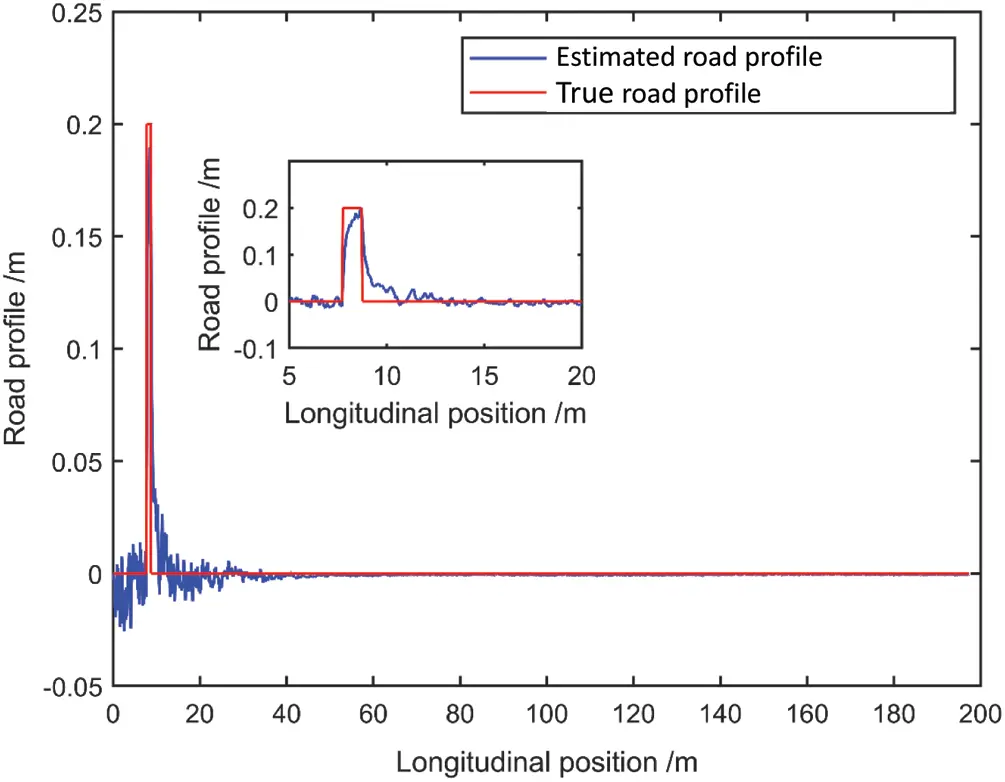Figure 6: The estimated and true road profiles (impact)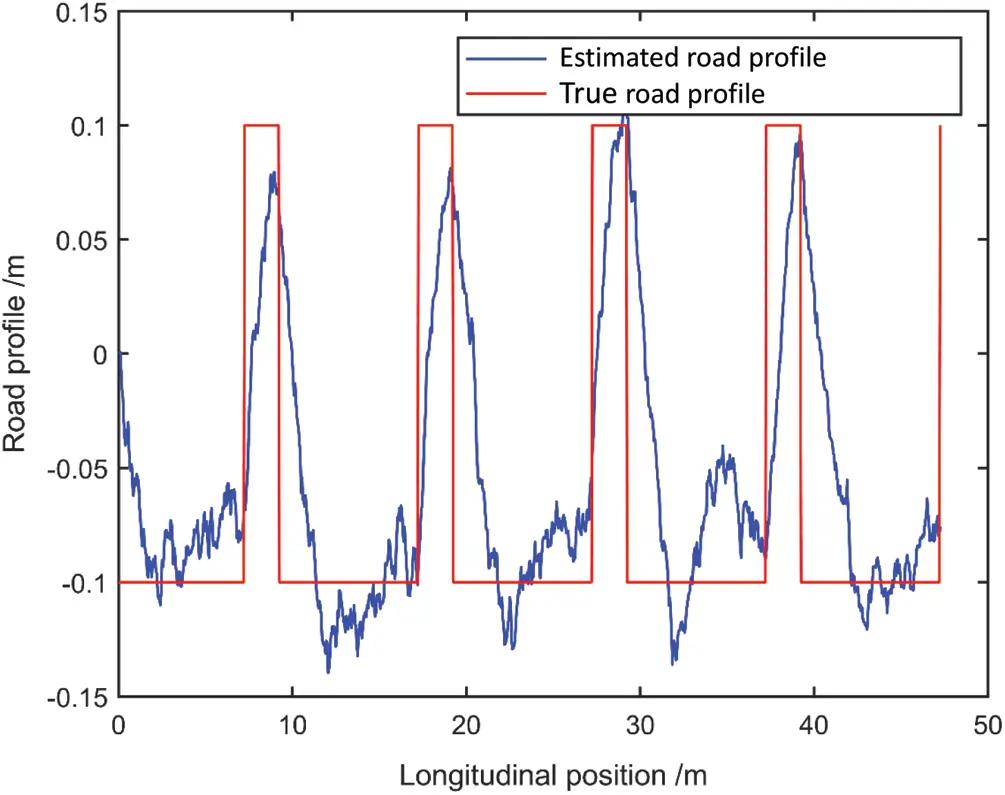Figure 7: Estimation in the presence of discrete obstacles

4.3 The Influence of the Vehicle Speed

It was pointed out in a previous study  that depending on the speed of the vehicle, a portion of the spatial frequency content of the road profile may be underestimated, and the estimation results of a vehicle at high speeds and low speeds are quite different.

However, via many numerical experiments, it was found that when keeping the other parameters unchanged, the calculation frequency of the Kalman filter can be adjusted appropriately, and similar estimated results can be obtained at different speeds. The approach is to keep the total computation time of the filter algorithm similar, i.e., to keep the spatial resolution constant. In this study, the sampling frequency was set to 1/20v (to ensure the maximum spatial frequency of the pavement spectrum), and the calculation time was set to L/v. For example, when the speed was set to 10 m/s, the frequency was set to 200 Hz; when the speed was set to 20 m/s, the frequency was set to 400 Hz (the total distance remained the same). The sampling frequency of the sensor is invariable in practical applications; thus, the resampling frequency of the algorithm must be changed according to the speed. Figs. 8 and 9 present the road profiles estimated at different speeds.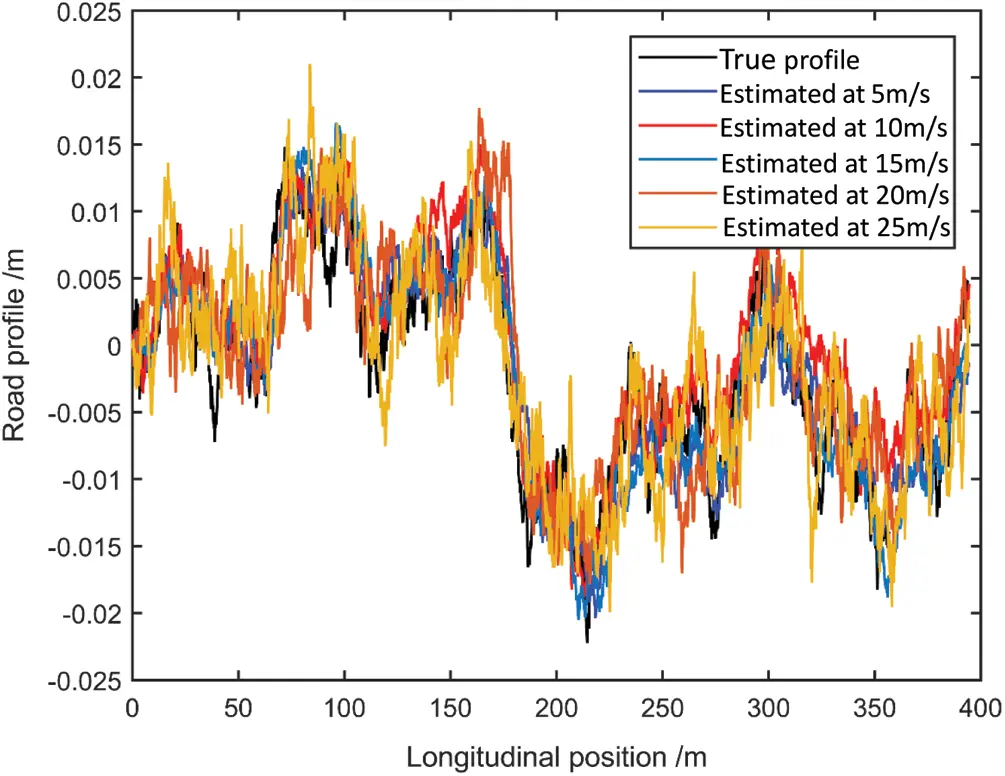Figure 8: The road profiles estimated at different speeds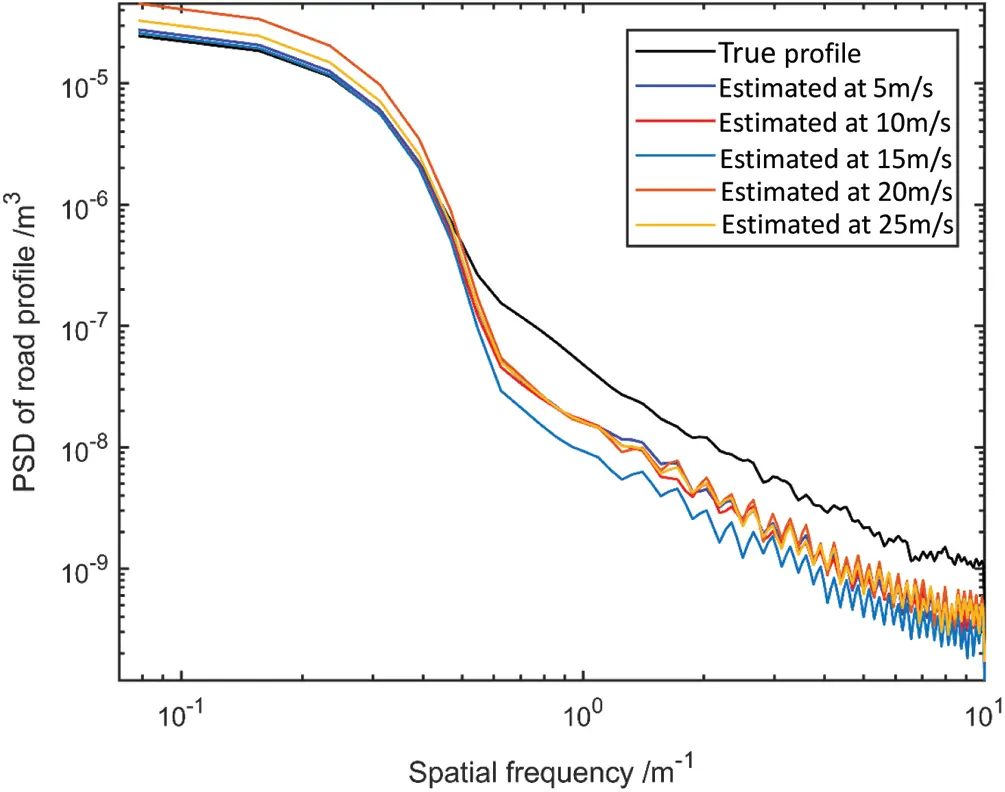Figure 9: The road profiles estimated at different speeds in terms of the PSD

After adjusting the speed algorithm, the proposed method can be summarized as a self-explanatory flowchart, as presented in Fig. 10.Figure 10: The flowchart of the proposed method

5  Experimental Study and Numerical Benchmark via Road Testing

A real vibration measurement test was carried out on a road with known parameters and various actual road conditions at different speeds. The results not only have important practical reference value, but can also provide an important benchmark for theoretical research.

To clearly present the test state, Fig. 11 illustrates the schematic diagram of the structure of the test vehicle and the sensor installation. There was a sliding support plate between the carrier and the carriage. A DHDAS dynamic signal acquisition and analysis system was used to record the vertical acceleration at four locations, as obtained by PCB 393B04 uniaxial accelerometers (with a measurement range of ±49 m/s2).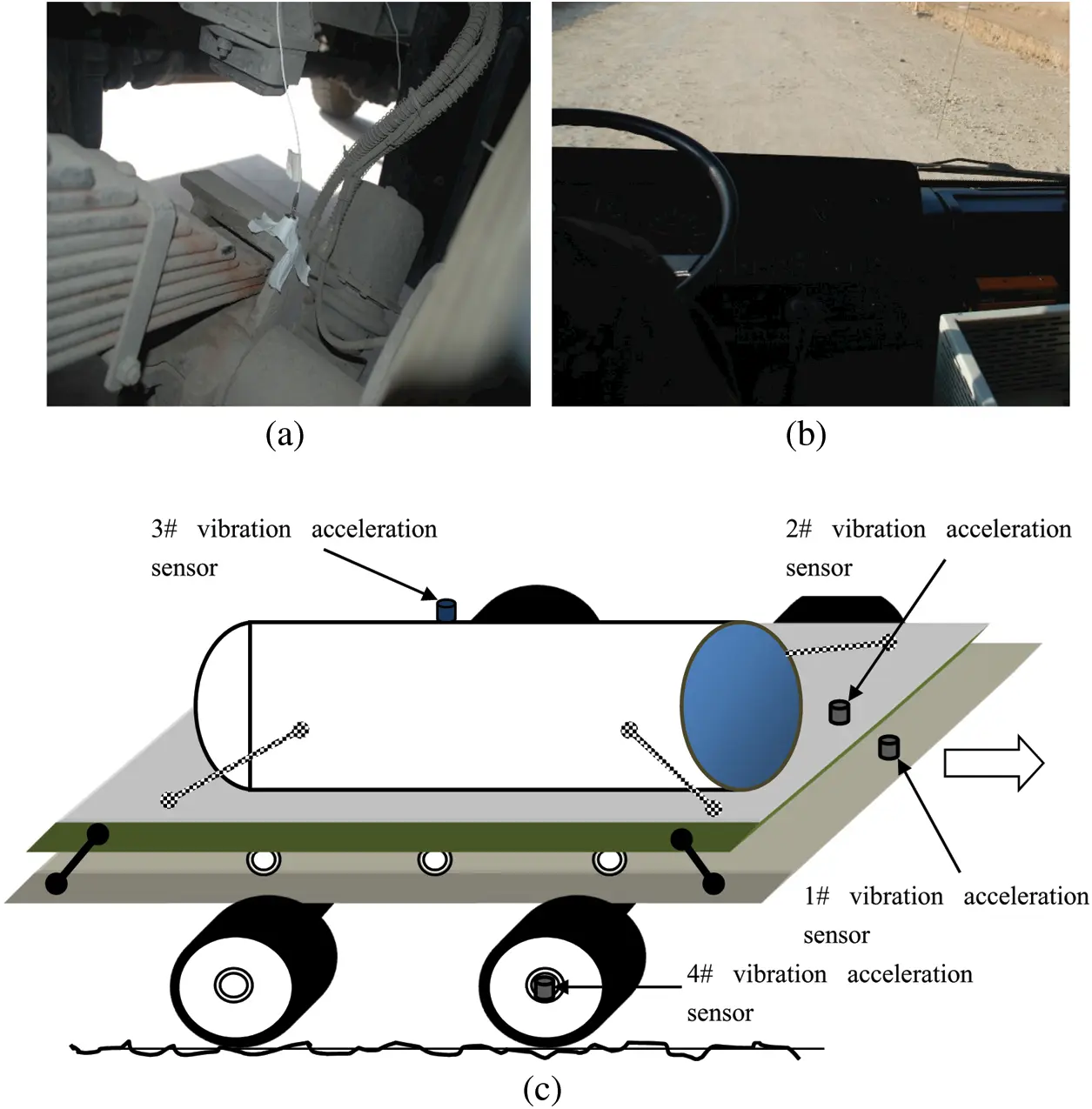Figure 11: The schematic diagram and photos of the vehicle driving vibration test. (a) Acceleration sensor (b) Test site (c) Sensor installation diagram

Two cases were considered, namely those at the speeds of 13.89 m/s (Case 1) and 9.72 m/s (Case 2). The sampling frequency was 5000 Hz, and each sampling period was 1 min. Because it was difficult to maintain a stable speed, only the time periods in which the speed was relatively stable were used for calculation. The acceleration responses of the four sensors in the two cases are respectively exhibited in Figs. 12 and 13.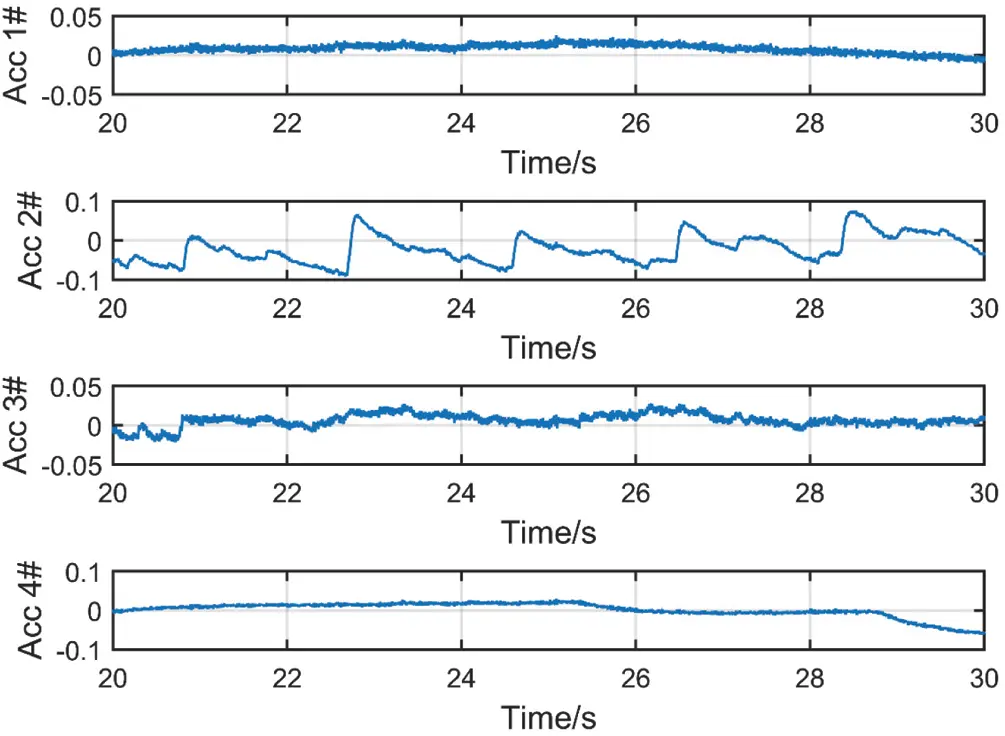Figure 12: The acceleration responses of the vehicle in Case 1 (unit: m/s2)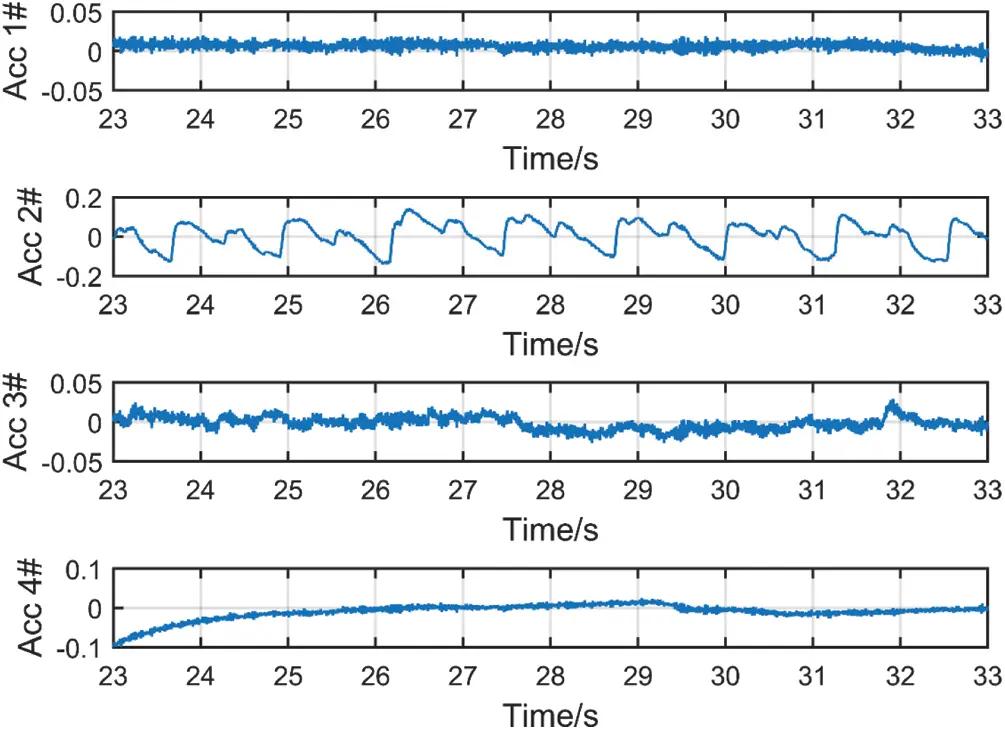Figure 13: The acceleration response of the vehicle in Case 2 (unit: m/s2)

The proposed method was then used to identify the pavement. It should be noted that the test data contained high levels of noise, which was filtered by the singular spectrum analysis (SSA) method proposed by Hossein et al. . Figs. 1417 present the estimation results of the two cases, and the RMSEs were 15.99% and 21.17%, respectively.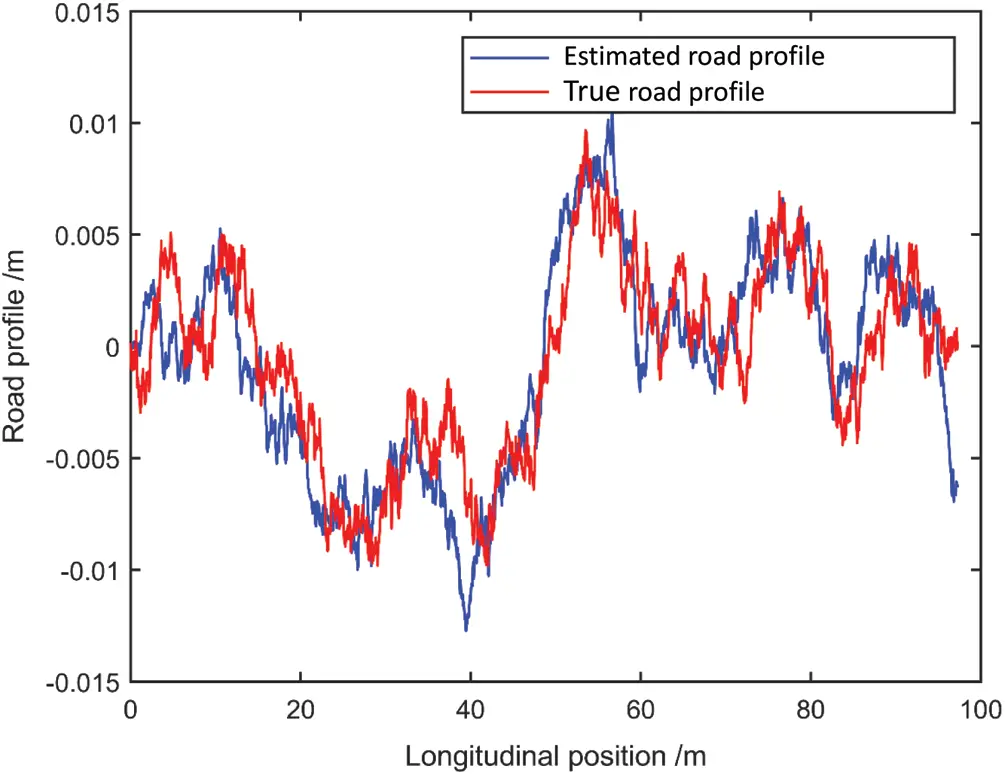Figure 14: The estimated and true road profiles (Case 1)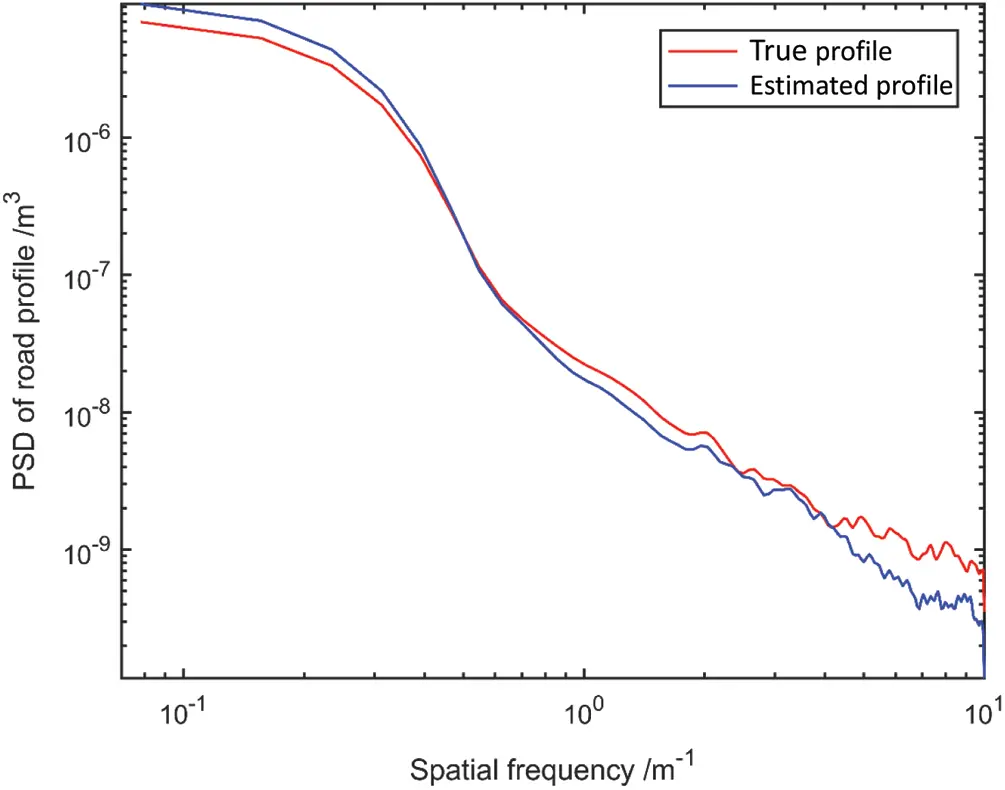Figure 15: The estimated and true road profiles in terms of the PSD (Case 1)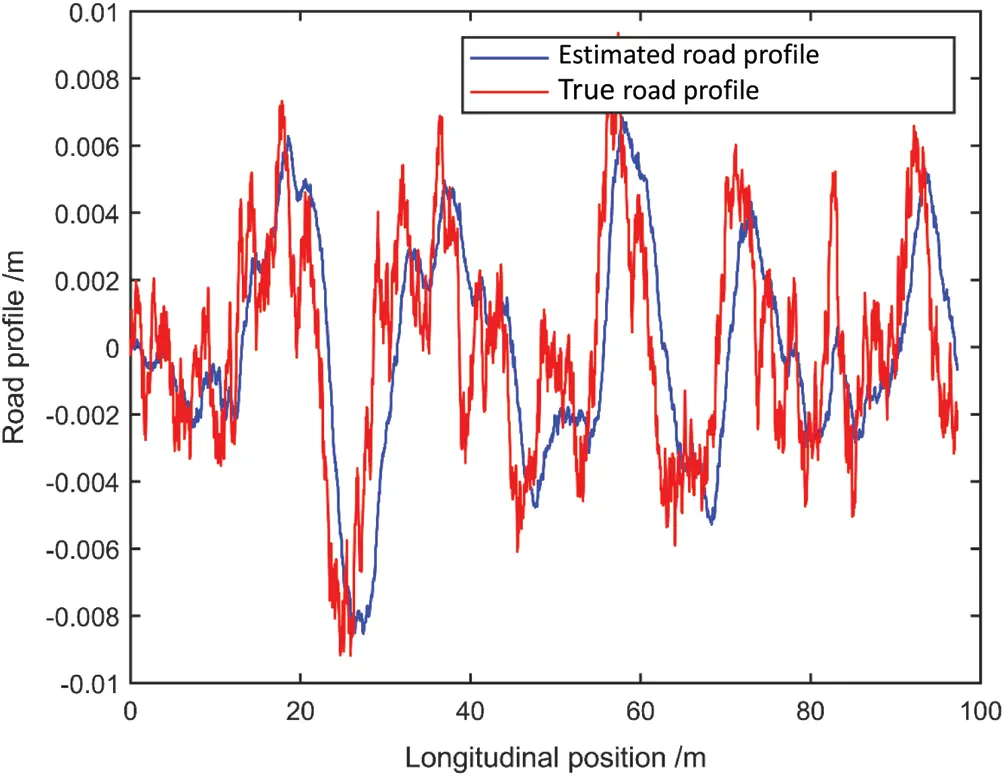Figure 16: The estimated and true road profiles (Case 2)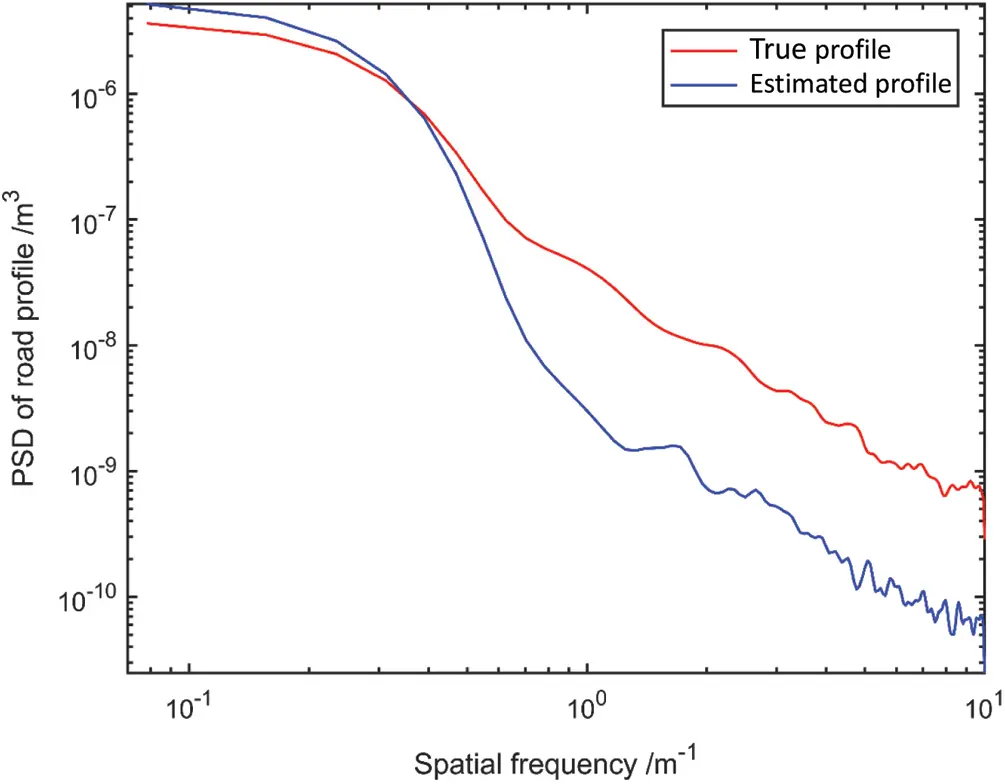Figure 17: The estimated and true road profiles in terms of the PSD (Case 2)

6  Conclusions

In this paper, a road profile estimation method based on the joint-input method was proposed. The obtained estimates may be used to predict load variability and calculate the vehicle responses, especially in the early stages of vehicle design. Moreover, to reduce the influence of the driving speed on the estimation results, a method of choosing the calculation frequency was proposed. To improve the stability of the algorithm, the precise integration method is used to calculate the algorithm parameters. The simulation results reveal that the RMSE of road roughness recognition was less than 10%, and the maximum error of discrete obstacle recognition was less than 15%. The effectiveness of the proposed method was also verified by experimental data.

In the future, the adaptive algorithm will be used to estimate corresponding parameters. The proposed method will also be applied in different driving situations (e.g., cornering, steering, accelerating, braking). It will also be applied to a nonlinear model, particularly a coupled car and road model.

Funding Statement: This work was supported by the Natural Science Foundation of Shaanxi Province (Grant No. 2021KW-25), the Astronautics Supporting Technology Foundation of China (Grant No. 2019-HT-XG), and the Fundamental Research Funds for the Central Universities (Grant No. 3102018ZY015).

Conflicts of Interest: The authors declare that they have no conflicts of interest to report regarding the present study.

## References

1. Poussot-Vassal, C., Sename, O., & Dugard, L. (2008). Attitude and handling improvements based on optimal skyhook and feedforward strategy with semi-active suspensions. International Journal of Vehicle Autonomous Systems, Special Issue on Modeling and Simulation of Complex Mechatronic Systems, 6(3–4), 308-329. [Google Scholar] [CrossRef]
2. Piasco, J. M., & Legeay, V. (1997). Estimation de l’uni longitudinal deschaussees par filtrage du signal del’analyseur de profil en long. Traitement du Signal, 14(4), 359-372. [Google Scholar]
3. Imine, H., Delanne, Y., & M’sirdi, N. K. (2005). Road profile inputs for evaluation of the loads on the wheels. Vehicle System Dynamics, 43(sup1), 359-369. [Google Scholar] [CrossRef]
4. Kim, H. J., Yang, H. S., & Park, Y. P. (2010). Improving the vehicle performance with active suspension using road-sensing algorithm. Computers and Structures, 80(18–19), 1569-1577. [Google Scholar] [CrossRef]
5. Yousefzadeh, M., Azadi, S., & Soltani, A. (2010). Road profile estimation using neural network algorithm. Journal of Mechanical Science and Technology, 24(3), 743-754. [Google Scholar] [CrossRef]
6. Solhmirzaei, A., Azadi, S., & Kazemi, R. (2012). Road profile estimation using wavelet neural network and 7-DOF vehicle dynamic systems. Journal of Mechanical Science and Technology, 26(10), 3029-3036. [Google Scholar] [CrossRef]
7. Ngwangwa, H. M., Heyns, P. S., Labuschagne, F. J. J., & Kululanga, G. K. (2010). Reconstruction of road defects and road roughness classification using vehicle responses with artificial neural networks simulation. Journal of Terramechanics, 47(2), 97-111. [Google Scholar] [CrossRef]
8. Burger, M. (2013). Calculating road input data for vehicle simulation. Multibody System Dynamics, 31(1), 93-110. [Google Scholar] [CrossRef]
9. Haddar, M., Baslamisli, S. C., Chaari, R., Chaari, F., & Haddar, M. (2019). Road profile identification with an algebraic estimator. Journal of Mechanical Engineering Science, 233(4), 1139-1155. [Google Scholar] [CrossRef]
10. Imine, H., Delanne, Y., & M’sirdi, N. (2006). Road profile input estimation in vehicle dynamics simulation. Vehicle System Dynamics, 44(4), 285-303. [Google Scholar] [CrossRef]
11. Gillijns, S., & de Moor, B. (2007). Unbiased minimum-variance input and state estimation for linear discrete-time systems with direct feedthrough. Automatica, 43(5), 934-937. [Google Scholar] [CrossRef]
12. Lourens, E., Reynders, E., de Roeck, G., Degrande, G., & Lombaert, G. (2012). An augmented Kalman filter for force identification in structural dynamics. Mechanical Systems and Signal Processing, 27(3), 446-460. [Google Scholar] [CrossRef]
13. Maes, K., Smyth, A. W., de Roeck, G., & Lombaert, G. (2016). Joint input-state estimation in structural dynamics. Mechanical Systems and Signal Processing, 70–71, 445-466. [Google Scholar] [CrossRef]
14. Lourens, E., Papadimitriou, C., Gillijns, S., Reynders, E., & de Roeck, G. (2012). Joint input-response estimation for structural systems based on reduced-order models and vibration data from a limited number of sensors. Mechanical Systems and Signal Processing, 29(4), 310-327. [Google Scholar] [CrossRef]
15. Fauriat, W., Mattrand, C., Gayton, N., Beakou, A., & Cembrzynski, T. (2016). Estimation of road profile variability from measured vehicle responses. Vehicle System Dynamics, 54(5), 585-605. [Google Scholar] [CrossRef]
16. Zhang, S., Deng, Z., & Li, W. (2007). A precise Runge-Kutta integration and its application for solving nonlinear dynamical systems. Applied Mathematics and Computation, 184(2), 496-502. [Google Scholar] [CrossRef]
17. Hassani, H., Soofi, A. S., & Zhigljavsky, A. A. (2010). Predicting daily exchange rate with singular spectrum analysis. Nonlinear Analysis: Real World Applications, 11(3), 2023-2034. [Google Scholar] [CrossRef]This work is licensed under a Creative Commons Attribution 4.0 International License, which permits unrestricted use, distribution, and reproduction in any medium, provided the original work is properly cited.